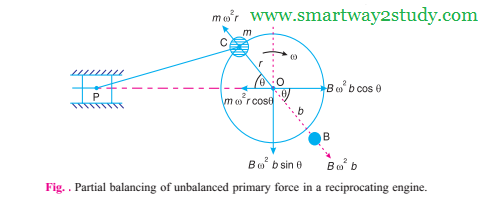Saturday, 26 August 2017

Partial Balancing of Unbalanced Primary Force in a reciprocating Engine

The primary unbalanced force may be considered as the component of the centrifugal force produced by a rotating mass 'm' placed at the crank radius 'r' as shown in below Fig.,The primary force act from O to P along the line of stroke. Hence, the balancing of primary force is considered as equivalent to the balancing of mass 'm' rotating at the crank radius 'r'. This is balanced by having a mass B at radius b, placed diametrically opposite to the crank pin C.
We know that centrifugal force due to mass B,
= B. w^2. b.
and horizantal component of this force acting in opposite direction of primary force
= B. w^2. b cos θ.
The primary force is balnced if
B. w^2. b cos θ = m w^2. r cos θ
or
B.b = m. r
A little consideration will show, that the primare force is completely balanced if B.b = m. r , but the centrifugal force produced due to the revolving mass B, has also a vertical  component perpendicular to the line of stroke having magnitude B. w^2. b sin θ. This force remains unbalnced. The maximum of this force occurs at value of θ = 90° or 270°
From the above discussion we know that there are two unbalced force, one along the line of stroke where as the second unbalnced force acts along the perpendicular to the line of stroke. The maximum valve of force remains same  in both the cases.It is thus obvious, that the effect of the above method of balancing is to change the direction of the maximum unbalnced force from the line of stroke to the perpendicular of line of stroke. A compromise let a fraction 'c' of the reciprocationg masses is balnced such that